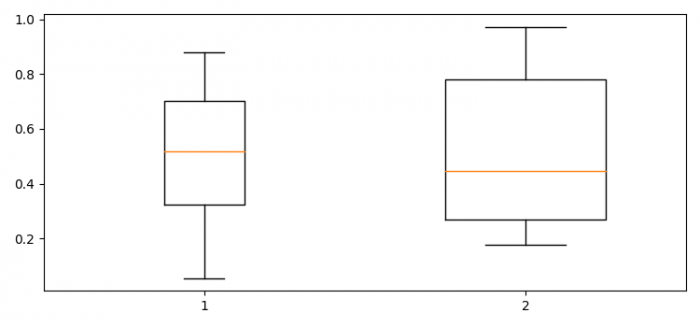# Adjust the width of box in boxplot in Python Matplotlib

MatplotlibPythonData Visualization

#### Python Data Science basics with Numpy, Pandas and Matplotlib

Most Popular

63 Lectures 6 hours

#### Data Visualization using MatPlotLib & Seaborn

11 Lectures 4 hours

#### MatPlotLib with Python

9 Lectures 2.5 hours

To adjust the width of box in boxplot in Python matplotlib, we can use width in the boxplot() method.

## Steps

• Set the figure size and adjust the padding between and around the subplots.
• Make a Pandas dataframe, i.e., two-dimensional, size-mutable, potentially heterogeneous tabular data.
• Make a box and whisker plot, using boxplot() method with width tuple to adjust the box in boxplot.
• To display the figure, use show() method.

## Example

import pandas as pd
import numpy as np
from matplotlib import pyplot as plt

plt.rcParams["figure.figsize"] = [7.50, 3.50]
plt.rcParams["figure.autolayout"] = True

data = pd.DataFrame({"Box1": np.random.rand(10), "Box2": np.random.rand(10)})
ax = plt.boxplot(data, widths=(0.25, 0.5))

plt.show()

## Output